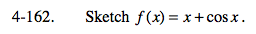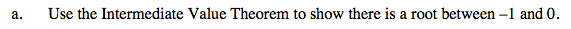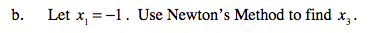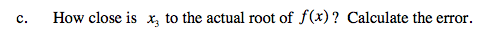### Home > CALC > Chapter 4 > Lesson 4.5.1 > Problem4-162

4-162.
1. Sketch f(x) = x + cos x. Homework Help ✎

1. Use the Intermediate Value Theorem to show there is a root between −1 and 0.

2. Let x1 = −1. Use Newton's Method to find x3.

3. How close is x3 to the actual root of f(x)? Calculate the error.State the conditions of the Intermediate Value Theorem: 'Since f(x) is continuous on the closed domain [−1, 0]...

Prove the thesis of the IVT: ... and f(−1) < 0 and f(0) > 0, there must be a value c, on −1 < c < 0, such that f(c) = 0.Check your work: x3 = −0.739x3 and the actual root should be very close.